TitleCollege Algebra
Tutorial 52: Solving Systems of Nonlinear Equations
in Two VariablesLearning Objectives

 After completing this tutorial, you should be able to: Solve a system of nonlinear equations in two variables by the substitution method. Solve a system of nonlinear equations in two variables by the elimination by addition method.Introduction

 In this tutorial we will be specifically looking at systems of nonlinear equations that have two equations and two unknowns.  We will look at solving them two different ways:  by the substitution method and by the elimination by addition  method.  These methods will be done in the same manner that they are done with systems of linear equations, we will just be applying it to nonlinear systems in this tutorial.  If you need a review on solving systems of linear equations in two variables, feel free to go to Tutorial 49: Solving Systems of Linear Equations in Two Variables.  So, let's go ahead and look at these systems.Tutorial

 System of Nonlinear Equations

 A system of nonlinear equations is two or more equations, at least one of which is not a linear equation, that are being solved simultaneously.  Note that in a nonlinear system, one of your equations can be linear, just not all of them. In this tutorial, we will be looking at systems that have only two equations and two unknowns.

 Solution of a System

 In general, a solution of a system in two variables is an ordered pair that makes BOTH equations true.  In other words, it is where the two graphs intersect, what they have in common.  So if an ordered pair is a solution to one equation, but not the other, then it is NOT a solution to the system. Since we are looking at nonlinear systems, in some cases, there may be more than one ordered pair that satisfies all equations in the system. A consistent system is a system that has at least one solution. An inconsistent system is a system that has no solution.   The equations of a system are dependent if ALL the solutions of one equation are also solutions of the other equation.  In other words, they end up being the same graph. The equations of a system are independent if they do not share ALL solutions.  They can have one point in common, just not all of them.

 There are three possible outcomes that you may encounter when working with these systems:

 Finite Number of Solutions If the system in two variables has one solution, it is an ordered pair that is a solution to BOTH equations.  In other words, when you plug in the values of the ordered pair it makes BOTH equations TRUE.  Since we are looking at nonlinear systems, in some cases there may be more than one ordered pair that satisfies all equations in the system. If you do get a finite number of solutions for your final answer, is this system consistent or inconsistent? If you said consistent, give yourself a pat on the back!  If you do get a finite number of solutions for your final answer, would the equations be dependent or independent? If you said independent, you are correct!   The graph below illustrates a system of two equations and two unknowns that has four solutions: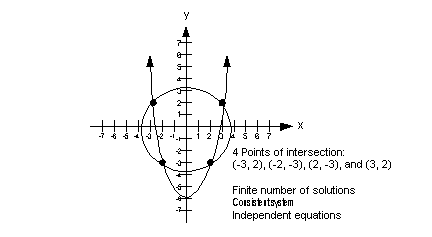No Solution In some cases, the equations in the system will not have any points in common.  In this situation, you would have no solution.   If you get no solution for your final answer, is this system consistent or inconsistent? If you said inconsistent, you are right!  If you get no solution for your final answer, would the equations be dependent or independent? If you said independent, you are correct!   The graph below illustrates a system of two equations and two unknowns that has no solution: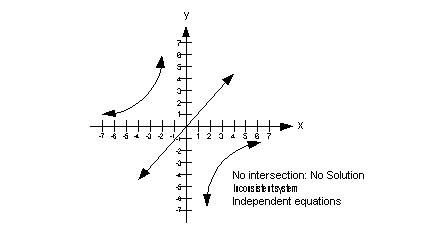Infinite Solutions If the two graphs end up lying on top of each other, then there is an infinite number of solutions.  In this situation, they would end up being the same graph, so any solution that would work in one equation is going to work in the other.   If you get an infinite number of  solutions for your final answer, is this system consistent or inconsistent? If you said consistent, you are right!  If you get an infinite number of  solutions for your final answer, would the equations be dependent or independent? If you said dependent, you are correct!   The graph below illustrates a system of two equations and two unknowns that has an infinite number of solutions: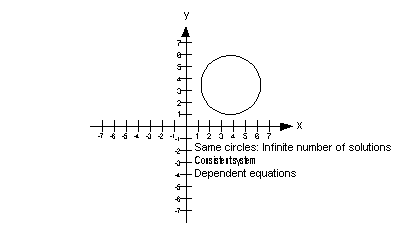Solve by the Substitution Method

 Step 1: Simplify if needed.

 This would involve things like removing ( ) and  removing fractions. To remove ( ): just use the distributive property.  To remove fractions: since fractions are another way to write division, and the inverse of divide is to multiply, you remove fractions by multiplying both sides by the LCD of all of  your  fractions.

 Step 2:  Solve one equation for either variable.

 It doesn't matter which equation you use or which variable you choose to solve for. You want to make it as simple as possible.  If one of the equations is already solved for one of the variables, that is a quick and easy way to go. If you need to solve for a variable, then try to pick one that has a 1 or -1 as a coefficient.  That way when you go to solve for it, you won't have to divide by a number and run the risk of having to work with a fraction (yuck!!). Also, it is easier to solve for a variable that is to the 1 power, as opposed to being squared, cubed, etc.

 Step 3: Substitute what you get for step 2 into the other equation.

 This is why it is called the substitution method.  Make sure that you substitute the expression into the OTHER equation, the one you didn't use in step 2. This will give you one equation with one unknown.

 Step 4:  Solve for the remaining variable.

 Step 5:  Solve for second variable.

 If you come up with a finite number of values for the variable in step 4, that means the two equations have a finite number of solutions.  Plug the value(s) found in step 4 into any of the equations in the problem and solve for the other variable.

 Step 6: Check the proposed ordered pair solution(s) in BOTH original equations.

 You can plug in the proposed solution(s) into BOTH equations.  If it makes BOTH equations true, then you have your solution to the system.  If it makes at least one of them false, you need to go back and redo the problem.Example 1:  Solve the system of equations by the substitution method: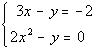Step 1: Simplify if needed.

 Both of these equations are already simplified.  No work needs to be done here.

 It does not matter which equation or which variable you choose to solve for.   Just keep it simple. Since the y in the first equation has a coefficient of -1, that would mean we would not run the risk of having to work with fractions (YUCK).  The easiest route here is to solve the first equation for y, and we definitely want to take the easy route.  You would not be wrong to either choose the other equation and/or solve for x, again you want to keep it as simple as possible. Solving the first equation for y we get: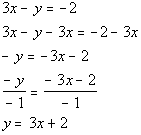*1st equation solved for y

 Substitute the expression 3x + 2 for y into the second equation and solve for x: (when you plug in an expression like this, it is just like you plug in a number for your variable)*Sub. 3x + 2 in for y *Dist. -1 through (  ) *Factor the trinomial   *Set 1st factor = 0           *Set 2nd factor = 0

 Step 5:  Solve for second variable.

 Plug in both values found for x in step 4.

 Plug in -1/2 for x into the equation in step 2 to find y's value.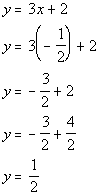*Plug in -1/2 for x

 (-1/2, 1/2) is one solution to this system.   Plug in 2 for x into the equation in step 2 to find y's value.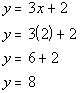*Plug in 2 for x

 (2, 8) is another solution to this system.

 You will find that if you plug either the ordered pair (-1/2, 1/2) OR (2, 8) into BOTH equations of the original system, that they are both a solution to BOTH of them. (-1/2, 1/2) and (2, 8) are both a solution to our system.Example 2:  Solve the system of equations by the substitution method: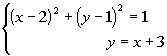Step 1: Simplify if needed.

 Even though we have ( ) in this problem, since we are going to do this by substitution, we do not need to clear them at this point to be able to do step 2.

 Note how the second equation is already solved for y.  We can use that one for this step. It does not matter which equation or which variable you choose to solve for.  But it is to your advantage to keep it as simple as possible.  Second equation solved for y: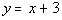*Solved for y

 Substitute the expression x + 3 for y into the first equation and solve for x: (when you plug in an expression like this, it is just like you plug in a number for your variable)*Sub. x + 3 for y *Square both binomials to clear the (   )           *Solve using the Square Root Method     *Solved for x

 Wait a minute, there is an i in the answer.  As mentioned above, in some cases we end up with no solution.  Here is an example where even though we came up with two values for x, we did not come up with a real number answer for a variable.  This means there is no solution.

 Step 5:  Solve for second variable.

 Since we did not get a real number for x, there is nothing to plug in here.

 There are no ordered pairs to check.   The answer is no solution.

 Solve by the Elimination by Addition Method

 Step 1: Simplify if needed.

 This would involve things like removing ( ) and  removing fractions. To remove ( ): just use the distributive property.  To remove fractions: since fractions are another way to write division, and the inverse of divide is to multiply, you remove fractions by multiplying both sides by the LCD of all of  your  fractions.

 Step 2: Multiply one or both equations by a number that will create opposite coefficients for like terms if needed.

 Add the two equations together. The variable that has the opposite coefficients will drop out in this step and you will be left with one equation with one unknown.

 Step 4: Solve for remaining variable.

 Solve the equation found in step 3 for the variable that is left. Most of the equations in this step will end up being either linear or quadratic.  Once in awhile you will run into a different type of equation. If you need a review on solving linear or quadratic equations, feel free to go to Tutorial 14: Linear Equations in On Variable or Tutorial 17: Quadratic Equations. Keep in mind that when you go to solve for this variable that you may end up with no solution for your answer.  For example, you may end up with your variable equaling the square root of a negative number, which is not a real number, which means there would be no solution. If both variables drop out and you have a FALSE statement, that means your answer is no solution.  If both variables drop out and you have a TRUE statement, that means your answer is infinite solutions, which would be the equation of the line.

 Step 5: Solve for second variable.

 If you come up with a finite number of values for the variable in step 4, that means the two equations have a finite number of solutions.  Plug the value(s) found in step 4 into any of the equations in the problem and solve for the other variable.

 Step 6: Check the proposed ordered pair solution(s) in BOTH original equations.

 You can plug the proposed solution(s) into BOTH equations.  If it makes BOTH equations true, then you have your solution to the system.  If it makes at least one of them false, you need to go back and redo the problem.Example 3:  Solve the system of equation by the elimination method: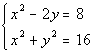Step 1: Simplify if needed.

 Both of these equations are already simplified.  No work needs to be done here.

 Again, you want to make this as simple as possible.  The variable that you want to eliminate must be a like variable.  In other words, it must not only be the same variable, but have the same exponent.  Note how x is squared on both equations.  So we would be able to get opposite coefficients on them and then when we added the two equations together they would drop out.  Note how y is to the one power in the first equation and squared on the second equation.  If we would pick y to eliminate we would have a problem because we cannot combine unlike terms together.  y squared -y would be y squared - y NOT 0. So I proposed that we multiply the second equation by -1, this would create a 1 and a -1 in front of the x squareds and we will have our opposites.   Multiplying the second equation by -1 we get: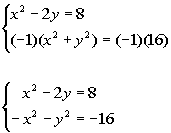*Mult. both sides of 2nd eq. by -1   *x squareds have opposite coefficients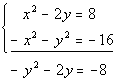*Note that x squareds dropped out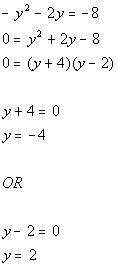*Set first factor = 0         *Set second factor = 0

 You can choose any equation used in this problem to plug in the found y values. I choose to plug in -4 for y into the first equation to find x's value.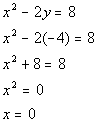*Plug in -4 for y

 So (0, - 4) is one solution to this system.

 I choose to plug in -4 for y into the first equation to find x's values.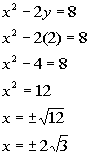*Plug in 2 for y   *Solve using the Square Root Method

 So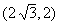and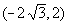are two more solutions to this system.

 You will find that if you plug the ordered pairs (0, - 4),, andinto BOTH equations of the original system, that they would all be solutions to BOTH of them. (0, - 4),, andare all solutions to our system.Example 4:  Solve the system of equation by the elimination method: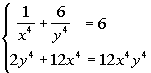Step 1: Simplify  if needed.

 The first equation is full of those nasty fractions.  We can simplify it by multiplying it by its LCD, just like you can do when you are working with one equation.  As long as you do the same thing to both sides of an equation, you keep the two sides equal to each other. Multiplying the first equation by its LCD we get: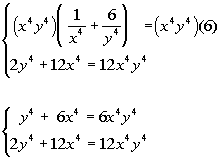*Multiply 1st eq. by its LCD x^4y^4

 Again, you want to make this as simple as possible.    The variable that you want to eliminate must be a like variable. Note that we could either choose to eliminate the x to the fourth terms or the y to the fourth terms or even the x to the fourth y to the fourth terms.  I'm going to choose to eliminate the x to the fourth terms, which means I want opposite coefficient on those terms.  Looks like we just need to multiply the first equation by -2. Multiplying the first equation by -2 we get: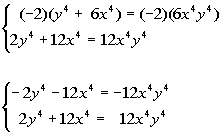*Mult. both sides of 1st eq. by -2     *x to the fourths have opposite coefficients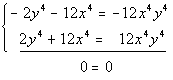*Note that everything dropped out

 Hey where did our variables go??  As mentioned above, if the variable drops out AND we have a TRUE statement, then when have an infinite number of solutions.  They end up being the same graph.

 There is no value to plug in here.

 There is no value to plug in here. When they end up being the same equation, you have an infinite number of solutions.  You can write up your answer by writing out either equation to indicate that they are the same equation. Two ways to write the answer are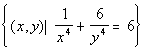OR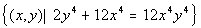.

 Special Note About Solving Nonlinear Systems Note that when solving these nonlinear systems, you may come across some that you are unable to use the substitution method  and some that you are unable to use the elimination by addition method.  Just try what you feel may work and if it doesn't work try the other method.Practice Problems

 These are practice problems to help bring you to the next level.  It will allow you to check and see if you have an understanding of these types of problems. Math works just like anything else, if you want to get good at it, then you need to practice it.  Even the best athletes and musicians had help along the way and lots of practice, practice, practice, to get good at their sport or instrument.  In fact there is no such thing as too much practice. To get the most out of these, you should work the problem out on your own and then check your answer by clicking on the link for the answer/discussion for that  problem.  At the link you will find the answer as well as any steps that went into finding that answer.Practice Problems 1a - 1c: Solve each system by either the substitution or elimination by addition method.

 1a.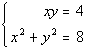(answer/discussion to 1a) 1b.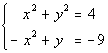(answer/discussion to 1b)

 1c.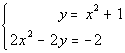(answer/discussion to 1c)Need Extra Help on these Topics?

 There were no good websites found to help us with the topics on this page.   Go to Get Help Outside the Classroom found in Tutorial 1: How to Succeed in a Math Class for some more suggestions.

Last revised on May 15, 2011 by Kim Seward.